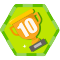## PANDAS 数据合并与重塑（concat篇） 原

pandas作者Wes McKinney 在【PYTHON FOR DATA ANALYSIS】中对pandas的方方面面都有了一个权威简明的入门级的介绍，但在实际使用过程中，我发现书中的内容还只是冰山一角。谈到pandas数据的行更新、表合并等操作，一般用到的方法有concat、join、merge。但这三种方法对于很多新手来说，都不太好分清使用的场合与用途。今天就pandas官网中关于数据合并和重述的章节做个使用方法的总结。

• 文中代码块主要有pandas官网教程提供。

# 1 concat

``````concat函数是在pandas底下的方法，可以将数据根据不同的轴作简单的融合
``````
``````pd.concat(objs, axis=0, join='outer', join_axes=None, ignore_index=False,
keys=None, levels=None, names=None, verify_integrity=False)``````

objs: series，dataframe或者是panel构成的序列lsit
axis： 需要合并链接的轴，0是行，1是列
join：连接的方式 inner，或者outer

## 1.1 相同字段的表首尾相接

``````# 现将表构成list，然后在作为concat的输入
In : frames = [df1, df2, df3]

In : result = pd.concat(frames)``````

``In : result = pd.concat(frames, keys=['x', 'y', 'z'])``

## 1.2 横向表拼接（行对齐）

### 1.2.1 axis

``In : result = pd.concat([df1, df4], axis=1)``

### 1.2.2 join

``In : result = pd.concat([df1, df4], axis=1, join='inner')``

### 1.2.3 join_axes

``In : result = pd.concat([df1, df4], axis=1, join_axes=[df1.index])``

## 1.3 append

``````append是series和dataframe的方法，使用它就是默认沿着列进行凭借（axis = 0，列对齐）
``````
``In : result = df1.append(df2)``

## 1.5 合并的同时增加区分数据组的键

### 1.5.1 可以直接用key参数实现

``In : result = pd.concat(frames, keys=['x', 'y', 'z'])``

### 1.5.2 传入字典来增加分组键

``````In : pieces = {'x': df1, 'y': df2, 'z': df3}

In : result = pd.concat(pieces)``````

## 1.6 在dataframe中加入新的行

append方法可以将 series 和 字典就够的数据作为dataframe的新一行插入。

``````In : s2 = pd.Series(['X0', 'X1', 'X2', 'X3'], index=['A', 'B', 'C', 'D'])

In : result = df1.append(s2, ignore_index=True)``````

## 表格列字段不同的表合并

``````如果遇到两张表的列字段本来就不一样，但又想将两个表合并，其中无效的值用nan来表示。那么可以使用ignore_index来实现。
``````

``````In : dicts = [{'A': 1, 'B': 2, 'C': 3, 'X': 4},
....:          {'A': 5, 'B': 6, 'C': 7, 'Y': 8}]
....:

In : result = df1.append(dicts, ignore_index=True)``````### 西伯利亚大尾巴狼Python数据分析(一) 关于pandas和numpy

2018/04/14
0
0
《利用Python进行数据分析》-数据规整

2019/08/06
0
0
[新手-数据分析师]pandas的学习笔记

pandas常用的函数有这些： pd.DataFrame pd.Series pd.read_csv pd.concat pd.Timestamp pd.to_datetime pd.date_range pd.index pd.merge 先从实际上能够用得到的函数开始学起走，然后遇到问...

inerds
2016/11/22
188
0
Python 专题区|数据分析工具 Pandas - 知乎

WISER Club数据科学杂谈
2019/10/21
0
0

2017/11/21
0
0

dwcz
6分钟前
21
0
WPF中的StaticResource和DynamicResource有什么区别？

javail
32分钟前
49
0
Day07继承中的面试题 答案

1. 每一个构造方法的第一条语句默认都是：super() Object类最顶层的父类。 class Zi extends Fu{ public int num = 20; public Zi(){ //super(); System.out.println("zi"); } 2.class Test......

Lao鹰
37分钟前
46
0

1 题目 Leetcode第18题,给定一个数组与一个target,找出数组中的四个数之和为target的不重复的所有四个数. 2 暴力 List<List<Integer>> result = new ArrayList<>();if (nums.length == 4 &......

Blueeeeeee
47分钟前
54
0
git clone --mirror和git clone --bare有什么区别

git clone帮助页面上有关于--mirror ： 设置远程存储库的镜像。 这意味着--bare 。 但没有详细介绍--mirror克隆与--bare克隆--mirror不同。 #1楼 克隆将从远程服务器复制参考，并将其填充到名...

72
0